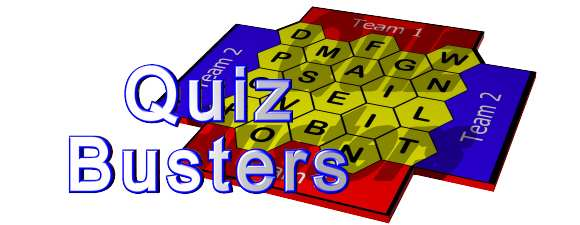# Quiz-Busters Games DirectorySearch for quizzes:
Subject:
Key Stage:

Found 35 results for Mathematics
Showing results 1 to 20
2 >>

Rounding Numbers (Key Stage 3 - Mathematics)
KS3 intro to rounding and decimal places.
Probability vocabulary (Key Stage 3 - Mathematics)
Key words / vocabulary used with the topic of probability and probability scales. Suitable for years 7 & 8.
Sequences (Key Stage 4 - Mathematics)
Sequences - keywords including diverging, converging, linear etc. Also some questions where they are given the nth term and need to work out a term. Good for GCSE revision.
Fractions and more (Key Stage 3 - Mathematics)
Fractions, Decimals and Percentages. Decimal Lengths
Using Brackets (Key Stage 2 - Mathematics)
Brackets involving addition, subtraction and multiplication.
Revision, spring 1 (Key Stage 2 - Mathematics)
Covers maths topics from spring 1, yr 3 of new strategy, eg. symmetry, shape and measure.
2D and 3D shapes (Key Stage 2 - Mathematics)
This quiz is about 2D and 3D shapes. It is aimed at year 3 pupils and can be used for a mental oral starter or plenary.
Length Weight Capacity (Key Stage 2 - Mathematics)
Revision of appropriate metric units to use when measuring length, weight and capacity.
Year 7 number work (Key Stage 3 - Mathematics)
Simple questions on rules for sequences, greater than and less than signs, maths words, directed numbers
Times Tables (x2 x3 x4 x5 x10) (Key Stage 2 - Mathematics)
Time Tables questions relating to the x2 x3 x4 x5 and x10 tables.
MMM and R (Key Stage 4 - Mathematics)
Mean, median, mode and range.
What number am i? (Key Stage 2 - Mathematics)
Numbers in words
Maths !!!! (Key Stage 4 - Mathematics)
General Questions on Mathematics GCSE Level
Block B - Unit 1 - Year 6 - quiz 1 (Key Stage 2 - Mathematics)
Block B - Unit 1 - Year 6 - Shape and Space to help learn maths vocabulary.
A1 - year 6 - quiz 1 (Key Stage 2 - Mathematics)
Quiz to help learn the new maths vocabulary for block A - unit 1 of year 6.
Basic sums (Key Stage 2 - Mathematics)
Quiz using basic addition, subtraction and multiplication to practice mental arithmetic.
Statistics (Key Stage 3 - Mathematics)
Statistical - common words used in data handling suitable for KS3.
Doubling and halving (Key Stage 1 - Mathematics)
Doubling and halving facts to 20.
Level E Angles and Lines (Key Stage 3 - Mathematics)
5-14 Level E Angles
Shapes (Key Stage 3 - Mathematics)
Year 7 Shapes and angles quiz. Suitable as a starter activity.

Showing results 1 to 20
2 >>